# Thread: Resistors in parallel

1. ## Resistors in parallel

Two variable resistors R1 and R2 are connected in parallel so that their combined resistance R is given by

1/R = 1/R1 + 1/R2

At an instant when R1 = 250 ohms and R2 = 1000 ohms, R1 is increatsing at a rate 100 ohms/minute. How fast must R2 be changing at that moment (a) to keep R constant? and (b) to enable R to increase at a rate of 10 ohms/minute?

--------------

Hello!

I have been sitting with the above exercise for a while now but cannot figure how to solve it. I assume I need to solve the derivative of R (or 1/R) for when it is 0, but cannot evet get that far. I would appreciate any help I can get.

Thanks!

2. Wow, that's a contrived problem.

Well, I think you're going to need to find the derivative of R with respect to t in terms of derivatives of R1 and R2 with respect to t. How do you propose to do that?

3. Yes! That was what I was trying to do :-).

Well. I only tried with (a) and I do not think it worked so well. Anyway, the derivative of the mentioned instant is 100 for R1. At first I thought I could say that the derivative of 1/R1 is then 1/100, but I suspect that is dead wrong.

But R = R1*R2/(R1+R2)

I now that the derivative of f(x)*g(x) = f'(x)*g(x) + f(x)*g'(x).

But from here I just get lostI have the derivative of R1, but not the function itself. It seems like I need to calculate the primitive function of it. Am I right? Would that be R1(t) = 250 + 100t? How would I do with R2?

I am confused, sorry.4. You have the correct equation for R. Now, I think you should go like this:

$\displaystyle R(t)=\frac{R_{1}(t)\,R_{2}(t)}{R_{1}(t)+R_{2}(t)}$
$\displaystyle R'(t)=\frac{(R_{1}(t)+R_{2}(t))(R_{1}(t)\,R_{2}(t) )'-(R_{1}(t)\,R_{2}(t))(R_{1}'(t)+R_{2}'(t))}{(R_{1}( t)+R_{2}(t))^{2}},$

and continue and simplify. Make sense?

5. It might be easier to differentiate the given equation implicitly, applying the chain rule to each of the 3 terms.

Just in case a picture helps...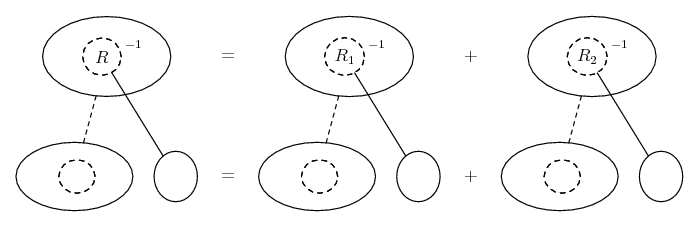or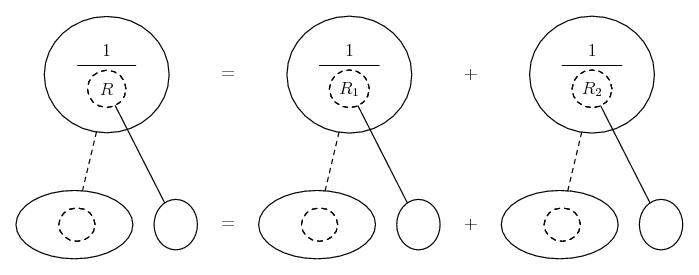... according to taste.

Where (key in spoiler)...

Spoiler:... is the chain rule. Straight continuous lines differentiate downwards (integrate up) with respect to t, and the straight dashed line similarly but with respect to the dashed balloon expression (the inner function of the composite which is subject to the chain rule).

Differentiate...

Spoiler: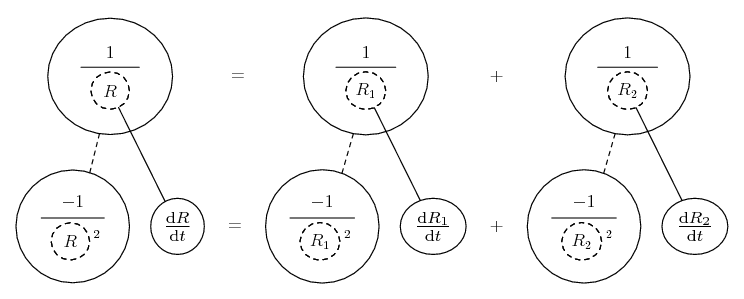Then plug in the values, including a zero derivative for R, for part (a)...

Spoiler: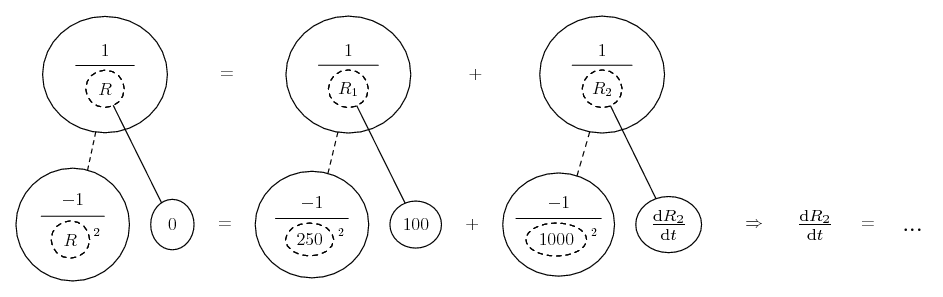Spoiler: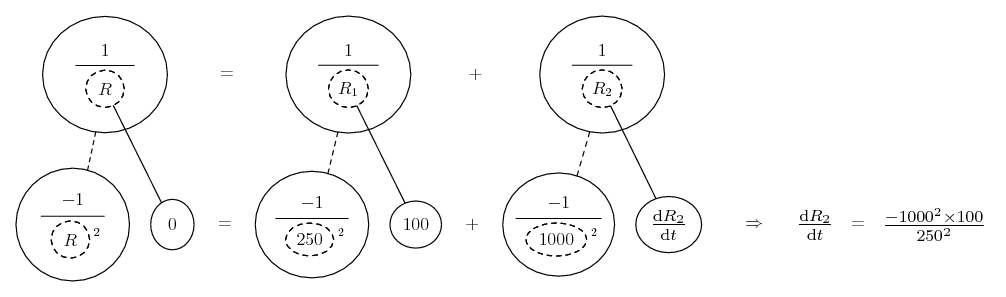... and using the formula to get a value for R in part b...

Spoiler: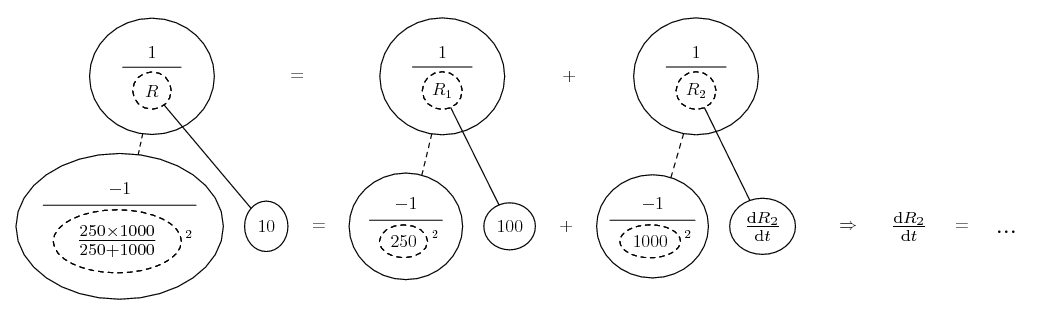_________________________________________

Don't integrate - balloontegrate!

Balloon Calculus; standard integrals, derivatives and methods

Balloon Calculus Drawing with LaTeX and Asymptote!

6. Ah, thanks. Now I understand. Thanks you both of you!

Fyi, I followed the path of Ackbeet, and evaluated the derivative of R to the same as he did, after some thinking. From that I got the expression:

$\displaystyle R_{2}'(t) = \frac{R_{1}(t)\,R_{2}(t)\,R_2{2}'(t)-R_{1}'(t)\,R_{2}'(t)^2}{R_{1}^2}$

and then

$\displaystyle R_{1}(t_{0}) = 250 + 100\,t_{0}$
$\displaystyle R_{2}(t_{0}) = 1000 + x\,t_{0}$

But that was a hell to calculate and does not seem to work.

7. I haven't checked your computations for the derivative of R_2, but you definitely can NOT assume that the resistors are linear in time.

For part a), I would plug in what you know, and then solve for what you don't know such that R'(t) = 0.

For part b), I would plug in what you know, and then solve for what you don't know such that R'(t) = 10.

Does that make sense?

8. I understand the solution Tom gave. To calculate the solution from the function for the derivative of R I have no clue of how to do, however. My intention was not really to assume it was linear in time. I understand that would not work. But I just thought I could make an approximation in that instant where R1 = 250 and R2 = 1000, to approximate the functions for R1 and R2 since the derivatives of R1 and R2 then would be 100 and x respectively. Is that approach really wrong?

9. I'm not sure I would call it "wrong" so much as "unnecessary". It's more work than the procedure I've outlined - moreover, I'm not so sure that's moving you closer to the solution of the problem, even if it's mathematically acceptable. Does that make sense? In other words, I think you're pursuing a tangent (pun intended).

10. In that case I do not know how to simplify the formula for R'.11. The target variable in both parts is $\displaystyle R_{2}'(t),$ right? If you start with my second equation in Post #4, and solve for $\displaystyle R_{2}'(t),$ what do you get? (Your answer in Post #6 is incorrect - try again. It's definitely going to have to have an $\displaystyle R'(t)$ in it!).

,

,

,

,

,

,

,

# differentiation of two parallel resistance

Click on a term to search for related topics.

#### Search Tags

derivative, extreme values, parallel, resistors, resistors in parallel